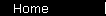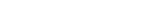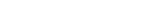# PEG Ratio

The PEG approach is a simple valuation tool, popularized by Peter Lynch and The Motley Fool among many others. Here is how Lynch puts it in One Up on Wall Street:

"The p/e ratio of any company that's fairly priced will equal its growth rate."

In other words,

P/E   =   G
where P/E is the stock's P/E ratio, and G is its earnings growth rate.

It looks simple and elegant, like a finance version of E = mc2, but watch out - this formula is strictly a rule of thumb, not a valid financial "law". (If you aren't convinced, just notice that the two sides of the formula have different units: you're comparing a fraction with a percent, meaning that a factor of 100 has magically appeared on one side only.)

So how accurate is this rule of thumb? It's certainly way off for at least some cases; for example, it implies that a company with zero growth should sell for a P/E of 0. But for normal values of growth stocks, this formula works surprisingly well. This calculator lets you compare the PEG approximation with the "correct" results from the cash flows calculator for different rates of "G":

 Annual Growth
Earnings Growth Rate:   %
 Results
Fair P/E Ratio
PEG Approximation:
Actual Value (via Discounted Cash Flows):

The assumptions are (1) a discount rate of 11%; (2) the growth rate you specify will be maintained for five years, after which growth will flatten out to zero.

If you do use this (or any other) valuation technique, remember to use it the way Lynch suggests: first find a company whose prospects seem attractive to you, and then use the techniques to make sure the price is reasonably attractive as well.

One way people misuse PEG and get themselves into trouble is by taking earnings from two successive years off of an annual report, and using them to calculate the earnings growth rate:

 Dangerous Calculator
This year's annual earnings per share: \$
Annual earnings per share from year(s) ago: \$

 Results
Annualized Earnings Growth Rate (over this period):   %
PEG Approximation for Fair P/E Ratio:

That's dangerous! Each year's earnings is a highly refined number, potentially including significant non-recurring items. That means the change between these two numbers can be very different from what you really want, namely, a conservative estimate for earnings growth that can be sustained over the next five years or more.

Finally, note that properly speaking the PEG ratio is defined as (P/E) / G. So the quote and formula from the top of the page are equivalent to saying that if a company is fairly priced, its PEG ratio ought to equal 1.0.

home  |  article  |  glossary  |  calculator  |  about us  |  booksValuation IntroA Little TheoryDCF CalculatorP/E RatioP/S RatioPEG RatioGraham FormulaDividend DiscountBuffett Formula (?)CAPM CalculatorBooks & LinksValuation FormulaStock Market PredictionsMeasuring Investment ReturnsStock Market CAGR